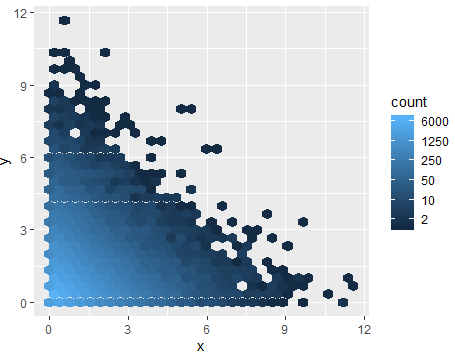4 views

Here's an example of a binned density plot:

require(ggplot2)

n <- 1e5

df <- data.frame(x = rexp(n), y = rexp(n))

p <- ggplot(df, aes(x = x, y = y)) + stat_binhex()

print(p)It would be nice to adjust the color scale so that the breaks are log-spaced, but a try

my_breaks <- round_any(exp(seq(log(10), log(5000), length = 5)), 10)

p + scale_fill_hue(breaks = as.factor(my_breaks), labels = as.character(my_breaks))

Results in an Error: Continuous variable () supplied to discrete scale_hue. Does it seem breaks is expecting a factor (maybe?) and designed with categorical variables in mind?

There's a not built-in workaround I'll post as an answer, but I think I might just be lost in my use of scale_fill_hue, and I'd like to know if there's anything obvious I'm missing.

by
edited

To build a logarithmic color scale in ggplot2, you can use the trans argument of scale_fill_gradient function and set the breaks as follows:

my_breaks = c(2, 10, 50, 250, 1250, 6000)

require("hexbin")

require(ggplot2)

n <- 1e5

df <- data.frame(x = rexp(n), y = rexp(n))

p <- ggplot(df, aes(x = x, y = y)) + stat_binhex()

p + scale_fill_gradient(name = "count", trans = "log",

breaks = my_breaks, labels = my_breaks)If you want to explore more in R programming then watch this R programming tutorial for beginner: